Open In App
Related Articles
• NCERT Solutions for Class 10 Maths

# Class 10 NCERT Solutions- Chapter 12 Areas Related to Circles – Exercise 12.2 | Set 2

### Question 8. A horse is tied to a peg at one corner of a square-shaped grass field of side 15 m by means of a 5 m long rope (see Fig.). Find

(i) the area of that part of the field in which the horse can graze.

(ii) the increase in the grazing area if the rope were 10 m long instead of 5 m. (Use π = 3.14)

Solution:

(i) Horse with graze=∅/(360°) × π × r2

= 90°/360° × 3.14 × 5 × 5

= 78.5/4

=19.625cm2

Area of circle the length of rope is increased to 10m

=∅/(360°) × π × r2

=90°/360° × 3.14 × 10 × 10

=314/4

=78.5cm2

(ii) Increasing in grazing area=78.5m2-19.635m2=58.875m2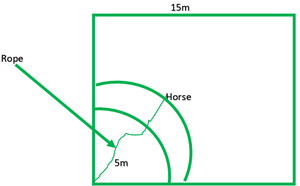### Question 9. A brooch is made with silver wire in the form of a circle with a diameter of 35 mm. The wire is also used in making 5 diameters which divide the circle into 10 equal sectors as shown in Fig. Find:

(i) the total length of the silver wire required.

(ii) the area of each sector of the brooch.

Solution:

(i) Total length of silver wire required=circumference of broach+5diameter

=5 × 35 mm × πd

=175+22/7 × 35

=175+110

=285mm

(ii) Area of each sector=1/10×Area of circle

=1/10×π×r2

=1/10×22/7×35/2×35×2

=11×35/4

=385/4mm2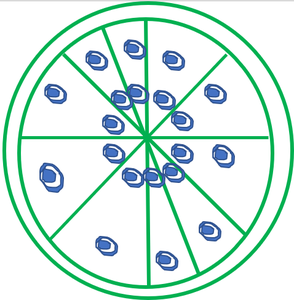### Question 10. An umbrella has 8 ribs that are equally spaced (see Fig.). Assuming the umbrella to be a flat circle of radius 45 cm, find the area between the two consecutive ribs of the umbrella.

Solution:

Total ribs in umbrella=8

Area between the two consecutive ribs=1/8π×r2

=1/8×22/7×45×45cm2

=22275/28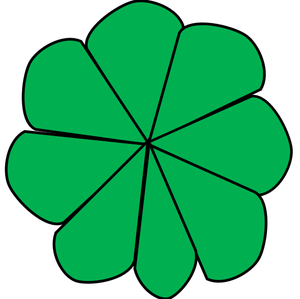### Question 11. A car has two wipers which do not overlap. Each wiper has a blade of length 25 cm sweeping through an angle of 115°. Find the total area cleaned at each sweep of the blades.

Solution:

r=25cm

Total area clean at each sweep of the blades=2×Area of sector

=2×∅/(360°)×π×r2

=2×(115°)/(360°)×22/7×25×25cm2

=23×11×25×25cm2

=158125/126cm2

=1254.96cm2

The total area clean at each sweep of blades=1254.96cm2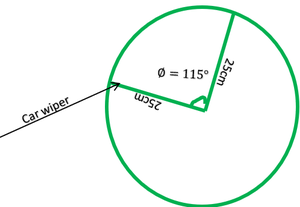### Question 12. To warn ships for underwater rocks, a lighthouse spreads a red colored light over a sector of angle 80° to a distance of 16.5 km. Find the area of the sea over which the ships are warned.

Solution:

(Use π = 3.14)

Distance over which light fall =r=16.5km

Area of the sea over which the ships are warned=Area of sector

=∅/(360°)×π×r2

=(80°)/(360°)×3.14×16.5×16.5

=1709.73

=189.97km2

The area of ships is +warned =189.97km2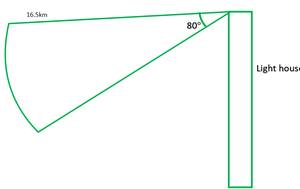### Question 13. A round table cover has six equal designs as shown in Figure. If the radius of the cover is 28 cm, find the cost of making the designs at the rate of ₹ 0.35 per cm2. (Use √3 = 1.7)

Solution:

Total equal designs==6

Cost for making design=RS.035 per cm2

∠O=360°/6=60°

Area of 1 design =Area of sector-Area of triangle

=∅/(360°)×π×r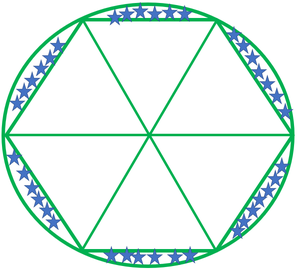### (A) p/180 × 2πR (B) p/180 × π R2 (C) p/360 × 2πR (D) p/720 × 2πR2

Solution:

Area of sector angle p=p/360×2πr2

Therefore, option (D) is correct.